SSC  >  Logical Reasoning - Test 3

# Logical Reasoning - Test 3

Test Description

## 25 Questions MCQ Test SSC CGL Tier 1 Mock Test Series | Logical Reasoning - Test 3

Logical Reasoning - Test 3 for SSC 2022 is part of SSC CGL Tier 1 Mock Test Series preparation. The Logical Reasoning - Test 3 questions and answers have been prepared according to the SSC exam syllabus.The Logical Reasoning - Test 3 MCQs are made for SSC 2022 Exam. Find important definitions, questions, notes, meanings, examples, exercises, MCQs and online tests for Logical Reasoning - Test 3 below.
Solutions of Logical Reasoning - Test 3 questions in English are available as part of our SSC CGL Tier 1 Mock Test Series for SSC & Logical Reasoning - Test 3 solutions in Hindi for SSC CGL Tier 1 Mock Test Series course. Download more important topics, notes, lectures and mock test series for SSC Exam by signing up for free. Attempt Logical Reasoning - Test 3 | 25 questions in 15 minutes | Mock test for SSC preparation | Free important questions MCQ to study SSC CGL Tier 1 Mock Test Series for SSC Exam | Download free PDF with solutions
 1 Crore+ students have signed up on EduRev. Have you?
Logical Reasoning - Test 3 - Question 1

### Directions: In the following question, select the related number from the given alternatives. 45 : 60 ∷ 120 : ?

Detailed Solution for Logical Reasoning - Test 3 - Question 1

In 45 : 60
45 = 9 × 5
60 = 10 × 6
Similarly;
120 = 12 × 10
143 = 13 × 11
Hence, “143” is correct option.

Logical Reasoning - Test 3 - Question 2

### In the following question, select the related letters from the given alternatives. FEGH : IJLK ∷ NMOP : ?

Detailed Solution for Logical Reasoning - Test 3 - Question 2

In FEGH : IJLK;
F + 3 = I; E + 5 = J; G + 5 = L;H + 3 = K
Similarly;
N + 3 = Q; M + 5 = R; O + 5 = T; P + 3 = S
Hence,

Logical Reasoning - Test 3 - Question 3

### In the following question, select the related word from the given alternatives. Chemist : Lab :: Students : ?

Detailed Solution for Logical Reasoning - Test 3 - Question 3

As chemist carries out his research in the lab likewise students study in the class.

Logical Reasoning - Test 3 - Question 4

Select the odd word from the given responses.

Detailed Solution for Logical Reasoning - Test 3 - Question 4

Dictionary, Novel and Journal is a type of book but Book itself can be Novel, Dictionary and Journal.
Hence, among all Book is odd one out.

Logical Reasoning - Test 3 - Question 5

Select the odd number from the given responses.

Detailed Solution for Logical Reasoning - Test 3 - Question 5

1. 5431 = 5 – 4 = 1; 4 – 3 = 1; 3 – 1 = 2
2. 9875 = 9 – 8 = 1; 8 – 7 = 1; 7 – 5 = 2
3. 7653 = 7 – 6 = 1; 6 – 5 = 1; 5 – 3 = 2
4. 6547 = 6 – 5 = 1; 5 – 4 = 1; 4 – 7 = -3
Here we can see that difference of last two digit is 2 in every option except d where the difference is 3.
Hence option “6547” is different from other responses

Logical Reasoning - Test 3 - Question 6

In the following questions, find the odd one out from the given alternatives.

Detailed Solution for Logical Reasoning - Test 3 - Question 6

Here the pattern followed is that each letter is increased by 2 than the previous one.

1. YZBDF = Y + 1 = Z;         Z + 2 = B;         B + 2 = D;        D + 2 = F

2. QSUWY = Q + 2 = S;         S + 2 = U;         U + 2 = W;       W + 2 = Y

3. TVXZB = T + 2 = V;         V + 2 = X;         X + 2 = Z;         Z + 2 = B

4. IKMOQ = I + 2 = K;          K + 2 = M;        M + 2 = O;       O + 2 = Q

In YZBDF, Z is only one more than Y hence it doesn’t follow the pattern and hence it is odd one out

Logical Reasoning - Test 3 - Question 7

Which one set of letters when sequentially placed at the gaps in the given letter series shall complete the given series?

_n m m n _n m n n _m n m n_

Detailed Solution for Logical Reasoning - Test 3 - Question 7
1. nn m m n nn m n n mm n m n= doesn’t form a pattern
2. nn m m n mn m n n nm n m n= doesn’t form a pattern
3. mn m m n nn m n n nm n m n= doesn’t form a pattern
4. nn m m / n mn m / n n mm / n m n= forms a perfect pattern

Hence, “n m m m” will complete the series.

Logical Reasoning - Test 3 - Question 8

A series is given with one term missing. Select the correct alternative from the given ones that will complete the series.

1, 1, 2, 12, ?

Detailed Solution for Logical Reasoning - Test 3 - Question 8

The followed pattern is:
First number = 1
(1 × 3) – 2 = 1
(1 × 6) – 4 = 2
(2 × 9) – 6 = 12
(12 × 12) – 8 = 136
Hence, 136 is the missing term.

Logical Reasoning - Test 3 - Question 9

In a certain code the following numbers are coded in a certain way be assigning signs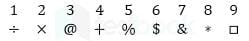Q. Which number can be decoded from the following?&%+□÷

Detailed Solution for Logical Reasoning - Test 3 - Question 9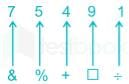Hence, “&%+□÷ “ will be coded as “75491”

Logical Reasoning - Test 3 - Question 10

If BE QUICK is coded as ZC OSGAI, then the code of last letter of third word in the sentence I LOVE MY COUNTRY is

Detailed Solution for Logical Reasoning - Test 3 - Question 10

Here,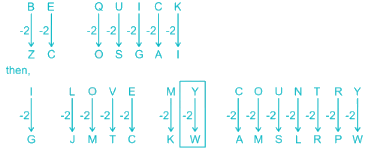Third Word is = MY.
Last letter of third word will be Y
Hence, “Y” will be coded as “W

Logical Reasoning - Test 3 - Question 11

A boy is facing East, then he turns left and goes 12 m, then turns right and goes 6 m, then goes 6 m to the South and from there 6 m to West. In which direction is he from his original place?

Detailed Solution for Logical Reasoning - Test 3 - Question 11

Boy is facing east and turns left i.eNorth .His path can be traced as:-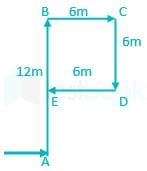A is the starting point and E is the ending point.
clearly, he is standing at north From the starting place A.

Logical Reasoning - Test 3 - Question 12

Arrange the following words in a dictionary order.

1. Pertinent
2. Persistent
3. Peracetate
4. Permanent
5. Perborate

Detailed Solution for Logical Reasoning - Test 3 - Question 12

After arranging as per the order of dictionary we get;
Peracetate, Perborate, Permanent, Persistent, Pertinent
Hence the correct order is 3, 5, 4, 2, 1.

Logical Reasoning - Test 3 - Question 13

Directions: Select the odd letter form the given responses.

Detailed Solution for Logical Reasoning - Test 3 - Question 13

1) ADBF = A + 3 = D; D – 2 = B; B + 4 = F
2) BECG = B + 3 = E; E – 2 = C;C + 4 = G
3) DHEI = D + 4 = H; H – 3 = E;E + 4 = I
4) MPNR = M + 3 = P; P - 2 = N; N + 4 = R
Hence, “DHEI” is different from other responses.

Logical Reasoning - Test 3 - Question 14

A word given in capital letters is followed by four words. Out of these only one cannot be formed by using the letters of the given word. Find out that word.

PRESTIGIOUS

Detailed Solution for Logical Reasoning - Test 3 - Question 14

1. PRESS = can be formed as PRESTIGIOUS.
2. PIOUS = can be formed as PRESTIGIOUS.
3. TIGER = can be formed as PRESTIGIOUS.
4. RIGID = cannot be formed as D is not present.
Hence “RIGID” is the correct option

Logical Reasoning - Test 3 - Question 15

In the following venn diagram, identify the politicians who are corrupt and cricket player.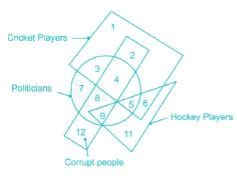Detailed Solution for Logical Reasoning - Test 3 - Question 15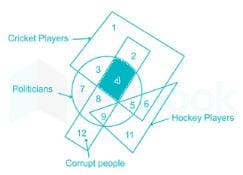Circle represents Politicians. Rectangle represents Corrupt people. Triangle represents Hockey players and Square represents Cricket players. Number which comes under circle square and rectangle represents politicians who are corrupt and cricket player which is equal to 4.

Logical Reasoning - Test 3 - Question 16

Directions: In each of the following questions, one/two statement/statement is/are given followed by two Assumptions/Conclusions I and II. You have to consider the statement to be true, even if it seems to be at variance from commonly known facts. You are to decide which of the given assumptions/conclusions can definitely be drawn from the given statement.

Statements:
All cars are bikes.
All bikes are ships.

Conclusions:
I. All cars are ships.
II. All ships are bikes.

Detailed Solution for Logical Reasoning - Test 3 - Question 16

representing the statements as Venn diagram:-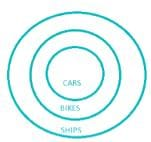I. All cars are ships = it definitely follows
II. All ships are bikes= it doesn’t follow since some ships are not bikes.
Hence, only conclusion I follows

Logical Reasoning - Test 3 - Question 17

Find the missing number from the given responses.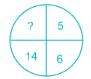Detailed Solution for Logical Reasoning - Test 3 - Question 17

Here in each column number in the left side = number in the right side × 2 + 2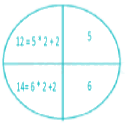Hence the missing number is 12

Logical Reasoning - Test 3 - Question 18

Directions: Study the following information carefully and answer the questions given below.

Seven boys P, Q, R, S, T, U and V are stand in a straight line facing north as follows.

1. R is standing between S and V.
2. Q is standing to the immediate left of T.
3. V is standing between R and Q.
4. U is Fourth left of V and S is immediate right of P.
Q. Who is standing exactly in the middle?

Detailed Solution for Logical Reasoning - Test 3 - Question 18

R is standing between S and V
⇒ S       R         V
Q is standing to the immediate left of T.
⇒ Q     T
V is standing between R and Q
⇒ R       V         Q
U is fourth left of V and S is immediate right of P
⇒ U P S R V
Combining all three situations we get,
⇒ U P S R V Q T
R is standing at the middle.

Logical Reasoning - Test 3 - Question 19

Directions: Study the following information carefully and answer the questions given below.

Seven boys P, Q, R, S, T, U and V are stand in a straight line facing north as follows.

1. R is standing between S and V.
2. Q is standing to the immediate left of T.
3. V is standing between R and Q.
4. U is Fourth left of V and S is immediate right of P.
Q. Who is standing to the extreme left?

Detailed Solution for Logical Reasoning - Test 3 - Question 19

R is standing between S and V
⇒ S       R         V
Q is standing to the immediate left of T.
⇒ Q     T
V is standing between R and Q
⇒ R       V         Q
U is fourth left of V and S is immediate right of P
⇒ U P S R V
Combining all three situations we get,
⇒ U P S R V Q T
U is standing at the extreme left.

Logical Reasoning - Test 3 - Question 20

DirectionsIn the following question, you have to identify the correct response from the given premises stated according to following symbols.

Q. If < represent ÷, > represent +, ^ represent –, × represent <, + represent >, - represent= and V represent x

Detailed Solution for Logical Reasoning - Test 3 - Question 20

As given in information: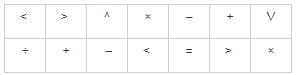1. (8 > 4 < 2 ^ 6 V 3 – 11) = (8 + 4 ÷ 2 – 6 × 3 = 11) = (-8 = 11) which is not true.
2. (8 ^ 4 < 2 > 6 V 3 + 11) = (8 – 4 ÷ 2 + 6 × 3 > 11) = (24 > 11) which is true.
3. (8 V 4 ^ 2 > 6 < 3 × 11) = (8 × 4 – 2 + 6 ÷ 3 < 11) = (32 < 11) which is not true.
4. (8 V 4 > 2 < 6 ^ 3 × 11) = (8 × 4 + 2 ÷ 6 – 3 < 11) = (29.33 <11) which is not true.

Option 2) satisfy the condition.

Logical Reasoning - Test 3 - Question 21

A square paper is folded in a particular manner and a punch is made. When unfolded, the paper appears as given below.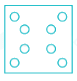Q. Find out the manner in which the paper is folded and punch is made from the responses given

Detailed Solution for Logical Reasoning - Test 3 - Question 21

Since one dot is present in every corner punch is made in the corner of the square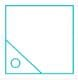Logical Reasoning - Test 3 - Question 22

Which answer figure will compete the question figure?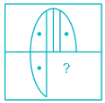Detailed Solution for Logical Reasoning - Test 3 - Question 22

option figure 4) completes the question figure as: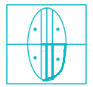Logical Reasoning - Test 3 - Question 23

How many rectangles are there which are not square in the given figure?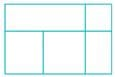Detailed Solution for Logical Reasoning - Test 3 - Question 23

Numbers of rectangle are shaded below:-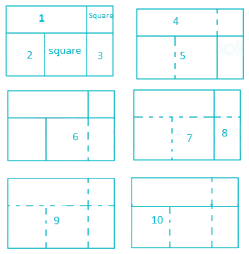Hence the number of rectangles are 10.

Logical Reasoning - Test 3 - Question 24

In question, one part of the figure is subtracted, choose the option that shows the correct result of subtraction (without the square box):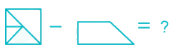Detailed Solution for Logical Reasoning - Test 3 - Question 24

Given image: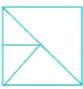If we remove this part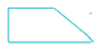We will get such: Dotted lines show removal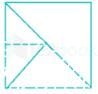Final image (without square box):Clearly, option 3 is the answer.

Logical Reasoning - Test 3 - Question 25

If a mirror is placed on the MN line, then which of the answer figures is the right image of the given figure?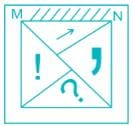Detailed Solution for Logical Reasoning - Test 3 - Question 25

Here the ‘arrow’ is at the top of the real image. So, it will come at the bottom of the mirror image. And the ‘question’ mark is at the bottom of the real image. So, it will come at the top of the mirror image. Since the mirror is places on the MN line, the ‘exclamation’ mark will remain in the left but its orientation will be opposite. Similar is the case of ‘comma’.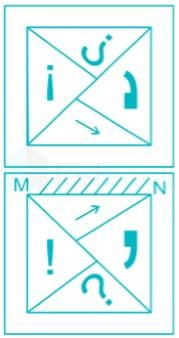## SSC CGL Tier 1 Mock Test Series

42 docs|103 tests
 Use Code STAYHOME200 and get INR 200 additional OFF Use Coupon Code
Information about Logical Reasoning - Test 3 Page
In this test you can find the Exam questions for Logical Reasoning - Test 3 solved & explained in the simplest way possible. Besides giving Questions and answers for Logical Reasoning - Test 3, EduRev gives you an ample number of Online tests for practice

## SSC CGL Tier 1 Mock Test Series

42 docs|103 tests